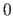Next: Matrix element Up: Fib elements Previous: Retrieve domain properties   Contents   Index

### Set-element

For the description of the set-element see section 9.12 on page.

The name of the set-XML element is `set`. It has an optional attribute named `domainNr`, which specifies the number of the domain for the set-element. If the attribute `domainNr` is missing, the domain number is.

The element contains first a XML element named `defined_variables`, wich contains for each variable, which the set-element defines, in their order, a `variable` element. These have the optinal attribute `number`, which is the number (counting begins at) of the element respectively variable defined in the set-element. Furthermore the `variable` element contains a natural number for the variable, that is not used above the set-element for another variable.

After the XML element for variable definitions `defined_variables` follows the XML element named `values`, wich contains the vectors with the values, to which the variables should be set. They are listed in their order in the set-element. The vectors all have the type `set` (`type=ßet"`).

At the end, after the XML element for values vectors `values`, the set-element contains the XML element for the contained the subobject.

An example for a set-element:

```<set>
<defined_variables>
<variable>7</variable>
<variable>8</variable>
<variable>9</variable>
</defined_variables>
<values>
<vector type=ßet">
<variable>1</variable>
<value>3</value>
<value>26.14</value>
</vector>
<vector type=ßet">
<value>33.4</value>
<value>-47</value>
<variable>4</variable>
</vector>
</values>

...<!-- Fib subobject -->
</set>
```

An example for a set-element with the optional attribute `domainNr` and `number`:

```<set domainNr="5">
<defined_variables>
<variable number="1">2</variable>
<variable number="2">3</variable>
<variable number="3">4</variable>
<variable number="4">5</variable>
</defined_variables>
<values>
<vector type=ßet">
<variable>1</variable>
<variable>1</variable>
<variable>1</variable>
<variable>1</variable>
</vector>
<vector type=ßet">
<value>8</value>
<value>4</value>
<variable>1</variable>
<value>3</value>
</vector>
</values>

...<!-- Fib subobject -->
</set>
```Next: Matrix element Up: Fib elements Previous: Retrieve domain properties   Contents   Index
Betti Österholz 2013-02-13